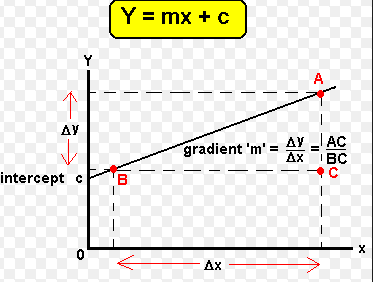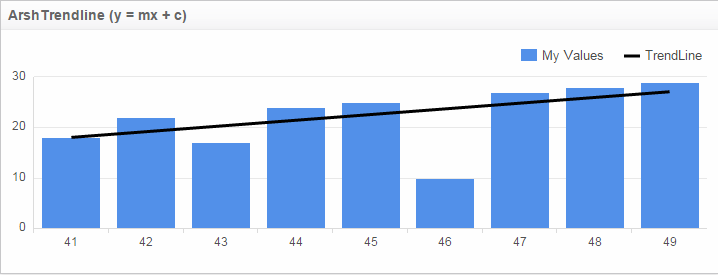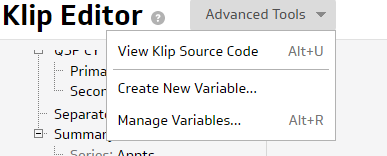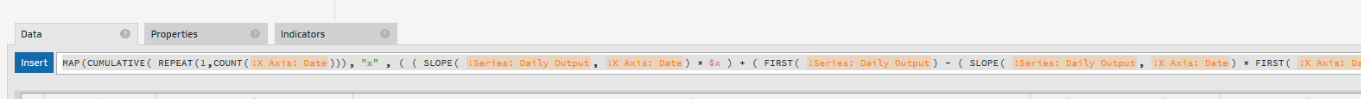The line formula is
Y = mX + c
where m is our Slope (slope = (y2 - y1/ x2 - x1))

and c is the y intercept.Here is how to add it in the Klip for any bar/line chart:

Black line is the Trendline:Formula :

( MAP( CUMULATIVE( REPEAT( 1 , COUNT( &series ) ) ) , "x" , ( ( SLOPE( &series , ARRAY( CUMULATIVE( REPEAT( 1 , COUNT( &series ) ) ) ) ) * \$x ) + ( FIRST( &series ) - ( SLOPE( &series , ARRAY( CUMULATIVE( REPEAT( 1 , COUNT( &series ) ) ) ) ) ) ) ) ) + offset )

'&series' is result reference to the Series formula.
'offset' is a minor adjustment that you may want to do in order to shift the line vertically.
"x" should be entered as string
x is a variable and can be created from Advanced Tools -> Create New Variable in the Klip editor:•The Data Girl

If you have dates or strings on the x-axis then you can use COUNT function in the MAP to achieve the trend line.•Jason Tran

Would you be able to provide the full formula for dates on the x-axis?

•Scott Salvesen

This is WAY too difficult, especially if you have dates on your X axis. Excel and Google both have a built-in function. Is there a way that we can get that here?

•Hi Jason, Scott,

The updated formula in the post. It doesn't refer the X-Axis anymore. So, if you have date as X-Axis, this should work fine.

•Mike Livingston

I'm having issues in that my trend line is always flat. It does change where it falls on the chart but I would expect it to slope one way or another. Any thoughts? Also, I'm not sure what variable x should be set to other than a variable.

•Chris Henry

The flat line still is an issue, as is the lack of explanation of what the "X" variable is supposed to do.  Any chance we can get updated instruction on this while we continue to wait for trend lines to be added as a feature?

•Hi Mike,

Could you please send the klip details to our support channel at support@klipfolio.com We'll have to check to check if there is anything missing in the formula or it needs to adjusted according to the data you have.

•Hi Chris,

Variable 'x' is part of MAP function to calculate the slope of the trendline. As explained in the post above, the formula for slope is y = mx + c.

To calculate each 'y' point, the variable 'x' is feeding the corresponding 'x' value ( these are not x-axis values but the x with respect to slope calculation ).

I have tested the formula which still works fine. Could you please share your klip details with our support team at support@klipfolio.com if you are seeing any issues.

Thanks,

•Ben Hoskins

Thanks - this is very helpful! This seems to be a Linear trend line (I think?) how would you go about making an exponential/power series trend line?

I also completely agree with the other post thread that this should be a standard "tick box" feature on graphs.